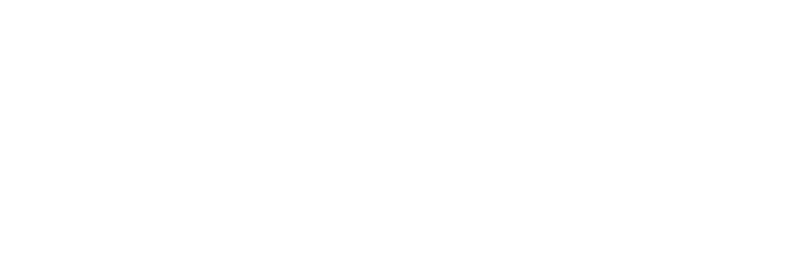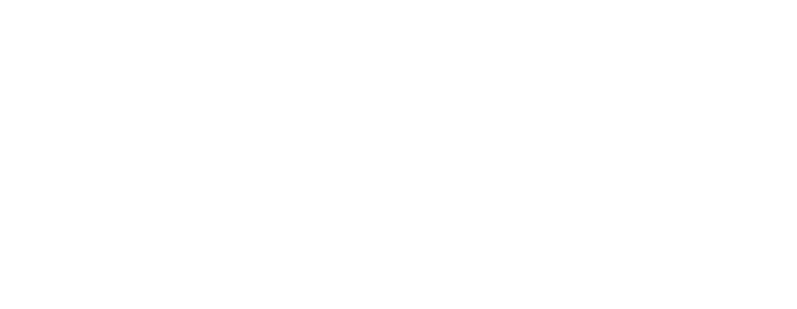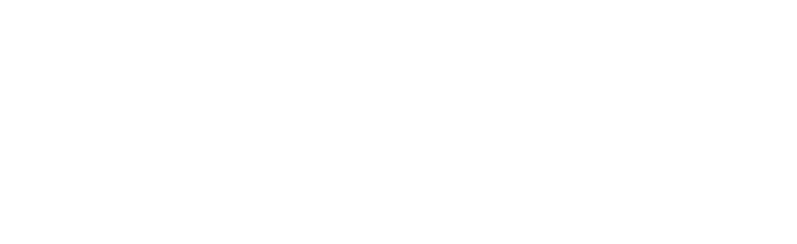# Data Visualization in R

## Visualization: Overview

The objective of this tutorial is to share with you a general overview of the plotting environments in R and of the most efficient way of coding your graphs in it. We will talk about the most important Integrated Development Environment (IDE) available for R as well as the most relevant packages available for plotting your data.

### Four Graphics Systems in R

There are currently 4 graphical systems available in R.

The base graphics system, written by Ross Ihaka, is included in every R installation.

The ‘grid’ graphics system, developed by Paul Murrell (2011), is implemented through the ‘grid’ package in R. ‘grid’ graphics provides a lower-level alternative to the standard graphics system. One key point to note here is that ‘grid’ graphics offers a lot of flexibility to the software developers, but lacks statistical graphics or complete plot.

The lattice package, developed by Deepayan Sarkar (2008), implements trellis graphs, as outlined by Cleveland (1985, 1993). So, trellis graphs display the distribution of a variable or the relationship between variables, separately for each level of one or more other variables. Built using the grid package, the lattice package provides a robust framework to visualizing multivariate data and a comprehensive alternative system for creating statistical graphics in R. There are many other packages like (effects, flexclust, Hmisc, mice and odfWeave) that use functions in the ‘lattice’ package to produce graphs.

Finally, the ggplot2 package, developed by Hadley Wickham (2009a), provides a system for creating graphs based on the grammar of graphics described by Wilkinson (2005) and expanded by Wickham (2009b). The intention of the ggplot2 package is to provide a comprehensive, grammar-based system for generating graphs in a coherent manner, allowing users to create new and innovative data visualizations. ggplot2 is one of the most celebrated packages in the realm of data visualisation because of the above-stated functionalities.

The lattice and ggplot2 packages overlap in functionality but approach the creation of graphs differently. Analysts tend to rely on one package or the other when plotting multivariate data. Given its power and popularity, the remainder of this tutorial will focus on ggplot2.

Let’s explore the ‘graphics’ package with some examples:

To generate the plot generated using graphics, use the following code:

plot(age~circumference, data=Orange)

The same graph can be generated using ggplot2 as well:

qplot(circumference, age, data=Orange)Generating box Plot using graphics and ggplot2:

boxplot(circumference~Tree, data=Orange)

To generate the plot using ggplot2, use the following code:

qplot(Tree, circumference, data=Orange, geom="boxplot")### ‘ggplot2’ – An introduction

As highlighted earlier, “The ggplot2 package basically implements a system for creating graphics in R based on a very comprehensive and coherent grammar.” In ggplot2 , the graphs are created by combining together functions using the “+” sign. Each function contributes to modify the plot created up to that point.

Let’s have a quick look at the following example:

ggplot(data=mtcars, aes(x=wt, y=mpg)) +

geom_point(pch=20, color="blue", size=2) +

geom_smooth(method="lm", color="purple", linetype=3) +

labs(title="Automobile Data", x="Weight", y="Mls Per Gallon")Let’s try to understand what ggplot does when it generates the graphics.

The ggplot() function first initializes the plot and specifies the data source (mtcars – in our example) and variables (wt, mpg) to be used. The options in the aes() function specify what role each variable will play. (aes stands for aesthetics, or how information is represented visually.) Here, the wt values are mapped along the x-axis, and mpg values are mapped along the y-axis. The ggplot() function here sets up the graph but produces no visual output on its own. Geometric objects (called geoms for short), which include points, lines, bars, box plots, and shaded regions, are added to the graph using one or more geom functions. In this example, the geom_point() function draws points on the graph, creating a scatter plot. The labs() function is optional and used for adding annotations (axis labels and a title).

Options to geom_point() set the point shape to circles (pch=20), double the points’ size (size=3), and render them in purple (color="purple"). The geom_smooth() function adds a “smoothed” line. Here a linear fit is requested (method="lm") and a purple dotted line (linetype=3) of size=2 is created. By default, the line includes 95% confidence intervals (the darker band).

The ggplot2 package provides methods for grouping and faceting. Grouping displays two or more groups of observations in a single plot. Groups are usually differentiated by color, shape, or shading. Faceting on the other hand displays groups of observations in separate, side-by-side plots. The ggplot2 package uses factors when they define groups or facets.

### Plot types in geoms

As the ggplot() function specifies the data source and variables to be plotted, the geom functions, on the other hand, decides how these variables are to be visually represented (using points, bars, lines, and shaded regions). Currently, 37 geoms are available. The following tables share the list of the most popular ones:

geom_bar()Bar Chartcolor, fill, alpha
geom_boxplot()Box Plotcolor, fill, alpha, notch, width
geom_density()Density Plotcolor, fill, alpha, linetype
geom_histogram()Histogramcolor, fill, alpha, linetype, binwidth
geom_jitter()Jittered Pointscolor, size, alpha, shape
geom_line()Line Graphcolorvalpha, linetype, size
geom_smooth()Fitted Linemethod, formula, color, fill, linetype, size
geom_text()Text AnnotationsMany; see the help for this function
geom_violin()Violin Plotcolor, fill, alpha, linetype
geom_point()Scatter Plotcolor, alpha, shape, size

Let’s look at one such example, which explores various options as stated above:

data(singer, package="lattice")

ggplot(singer, aes(x=voice.part, y=height)) +

geom_violin(fill="lightblue") +

geom_boxplot(fill="lightgreen", width=.1)The above code snippet shows how you can combine two different graph types (box plot and violin plot) two create a new one. The box plots show the 25th, 50th, and 75th percentile scores for each voice part in the singer data frame, along with any outliers. The violin plots provide more visual cues as to the distribution of scores over the range of heights for each voice part.

### Grouping

In order to develop a better understanding of the data, it is often required to plot two or more groups of observations together in the same graph. Grouping is accomplished in ggplot2 graphs by associating one or more grouping variables with visual characteristics such as shape, color, fill, size, and line type.

Let’s use grouping functionality to explore the Salaries dataset. The data frame contains information on the salaries of university professors collected during the period 2008–2009 (academic year). Variables include rank (AsstProf, AssocProf, Prof), sex (Female, Male), yrs.since.phd (years since Ph.D.), yrs.service (years of service), and salary (nine-month salary in dollars) etc.

require(carData)

data(Salaries, package="carData")

library(ggplot2)

ggplot(data=Salaries, aes(x=salary, fill=rank)) +geom_density(alpha=.7)One can also visualize the number of professors by their rank and some other attributes (sex) using a grouped bar chart. For example:

ggplot(Salaries, aes(x=rank, fill=sex)) + geom_bar(position="stack") + labs(title='arrangement="stack"')Alternatively you can use other types of position values (position=’dodge’ or position=’fill’)

For example:

ggplot(Salaries, aes(x=rank, fill=sex)) + geom_bar(position="fill") + labs(y = "Proportion",title='arangement="fill"')Each of the plots emphasizes different aspects of the data. These graphs reveal different insights about the data like there are more female full professors than a female assistant or associate professors or the 2nd chart shows that the relative percentage of women to men in the full-professor group is less than in the other two groups, even though the total number of women is greater.

### Faceting

Sometimes it becomes easier to demonstrate the relationships if the groups appear in side-by-side graphs (called faceted graphs in ggplot2). You can create faceted graphs by using facet_wrap() and facet_grid() functions.

The table below shows a list of the facet functions in ggplot2:

SyntaxResults
facet_wrap(~var, ncol=n)Separate plots for each level of var arranged into n columns
facet_wrap(~var, nrow=n)Separate plots for each level of var arranged into n rows
facet_grid(rowvar~.)Separate plots for each level of rowvar, arranged as a single column
facet_grid(rowvar~.)Separate plots for each level of rowvar, arranged as a single column

Let’s look at one example:

data(singer, package="lattice")

library(ggplot2)

ggplot(data=singer, aes(x=height)) +

geom_histogram() +

facet_wrap(~voice.part, nrow=4)The resulting plot displays the distribution of singer heights by voice part. Separating the eight distributions into their own small, side-by-side plots makes them easier to compare.

Another example:

data(singer, package="lattice")

library(ggplot2)

ggplot(data=singer, aes(x=height, fill=voice.part)) +

geom_density() +

facet_grid(voice.part~.)This chart is displaying the height distribution of choral members in the singer dataset separately for each voice part, using kernel-density plots arranged horizontally.

Let’s look at a few other examples of the application of ggplot2:

set.seed(321) #for reproducibility

x <-data.frame(x=rnorm(10000)) #Generating a random data points

ggplot(data=x, aes(x=x)) +

geom_histogram(aes(y=..density..,fill=..density..)) +

geom_density()In this example, we just created a simple normal distribution with default values (0 as the mean and 1 as the standard deviation) using the rnorm function, and then we used them to create a histogram of such a distribution. We can then map the filling color to the number of observations in each bin available in the new count variable created by the stat_bin() function. Just remember that, in order to avoid errors because of variables with the same name in the original dataset, the newly created variables must be surrounded by .., so in our example, we would need to use ..count...

Applying this method to aesthetic mapping, we use a continuous scale of color tones to map the observation count. Since the scale is continuous, we cannot apply this method on geometries with only one continuous plot area, such as geom_density(), which generate a smooth estimate of the kernel density. On the other side, you can apply it to the histogram representing the density of observations. One can, in fact, use the new variable density created by the stat_bin() function to represent as a y value for the density of observations present in each bin and at the same time use a filling color proportional to the observations. The above code snippet does exactly the same thing.

ggplot(data=x, aes(x=x)) + geom_histogram(aes(alpha=..count..))This is a histogram of a normally distributed random variable representing the data count with transparency value (alpha) mapped to the data count.

ggplot(data=x, aes(x=x)) +

geom_histogram(aes(alpha=..count..,fill=..count..))This is exactly the same plot as the previous one but also includes a filling mapping to the data count.

We can also add text and references line for a graph:

Example code:

ggplot(x, aes(x=x)) +

geom_histogram(alpha=0.7) +

geom_vline(aes(xintercept=median(x)), color="green", linetype="dashed",

size=1) +

geom_hline(aes(yintercept=40), col="red", linetype="solid") +

geom_text(aes(x=median(x),y=90),label="Median",hjust=1) +

geom_text(aes(x=median(x),y=90,label=round(mean(x),

digit=3)),hjust=-0.7)The ggplot2 package offers a wide range of functions for calculating various statistical summaries that can be added to graphs. These include functions for binning data and calculating densities, contours, and quantiles. This section looks at methods for adding smoothed lines (linear, nonlinear, and nonparametric) to scatter plots.

For example, You can use the geom_smooth() function to add a variety of smoothed lines and confidence regions. An example of a linear regression with confidence limits was given in the following images:

data(Salaries, package="carData")

library(ggplot2)

ggplot(data=Salaries, aes(x=yrs.since.phd, y=salary)) +

geom_smooth() + geom_point()The plot suggests that the relationship between experience and salary isn’t linear, at least when considering faculty who graduated many years ago. As an alternative approach, next, let’s fit a quadratic polynomial regression (one bend) separately by gender:

ggplot(data=Salaries, aes(x=yrs.since.phd, y=salary,

linetype=sex, shape=sex, color=sex)) +

geom_smooth(method=lm, formula=y~poly(x,2),

se=TRUE, size=1) +

geom_point(size=1)

The confidence limits are also displayed to simplify the graph (se=TRUE). Genders are differentiated by color, symbol shape, and line type.Apart from these, there are many other functionalities you can invoke to make the graphs look richer like (axes, legends, scales, themes etc.)

The number of functionalities is quite huge for ggplot2. It is a very rich package with way too many options to play around. But the encouraging part is that wealth of material is available to help you out. A list of all ggplot2 functions, along with examples, can be found at http://docs.ggplot2.org.

In this tutorial, we tried to cover major aspects related to R-graphics with a key focus on the ggplot2.R

## Swift Tutorial

Introduction to Swift Tutorial

## C# Tutorial

C# is an object-oriented programming developed by Microsoft that uses the .Net Framework. It utilizes the Common Language Interface (CLI) that describes the executable code as well as the runtime environment. C# can be used for various applications such as web applications, distributed applications, database applications, window applications etc.For greater understanding of this tutorial, a basic knowledge of object-oriented languages such as C++, Java etc. would be beneficial.
9128
C# Tutorial

C# is an object-oriented programming developed by Microsoft that uses ...